Question

# Multisim simulation of colpitts oscillator (using opamp) for frequency 100MHz

Multisim simulation of colpitts oscillator (using opamp) for frequency 100MHz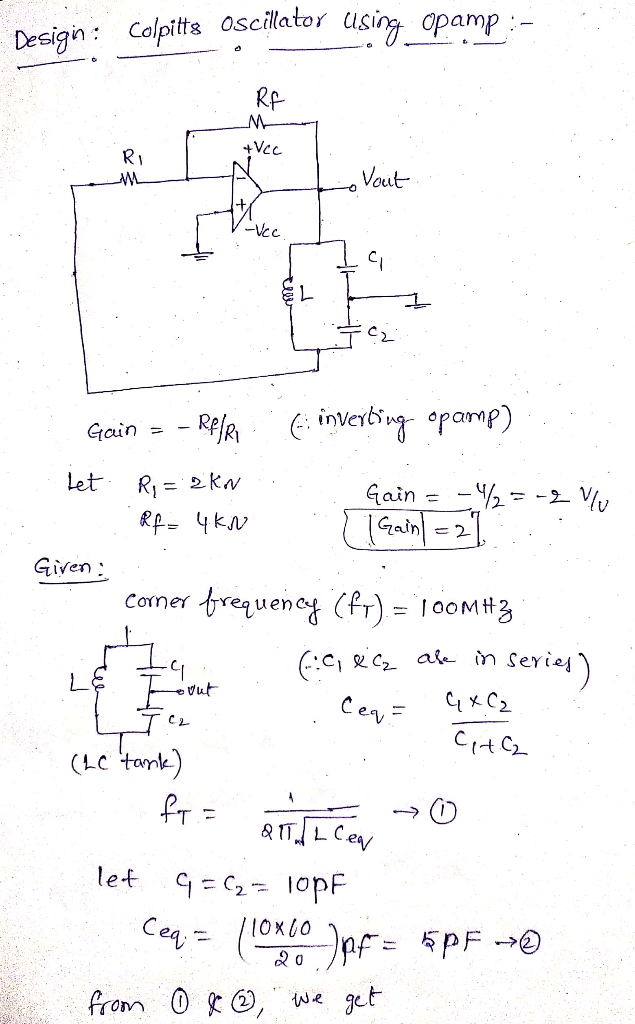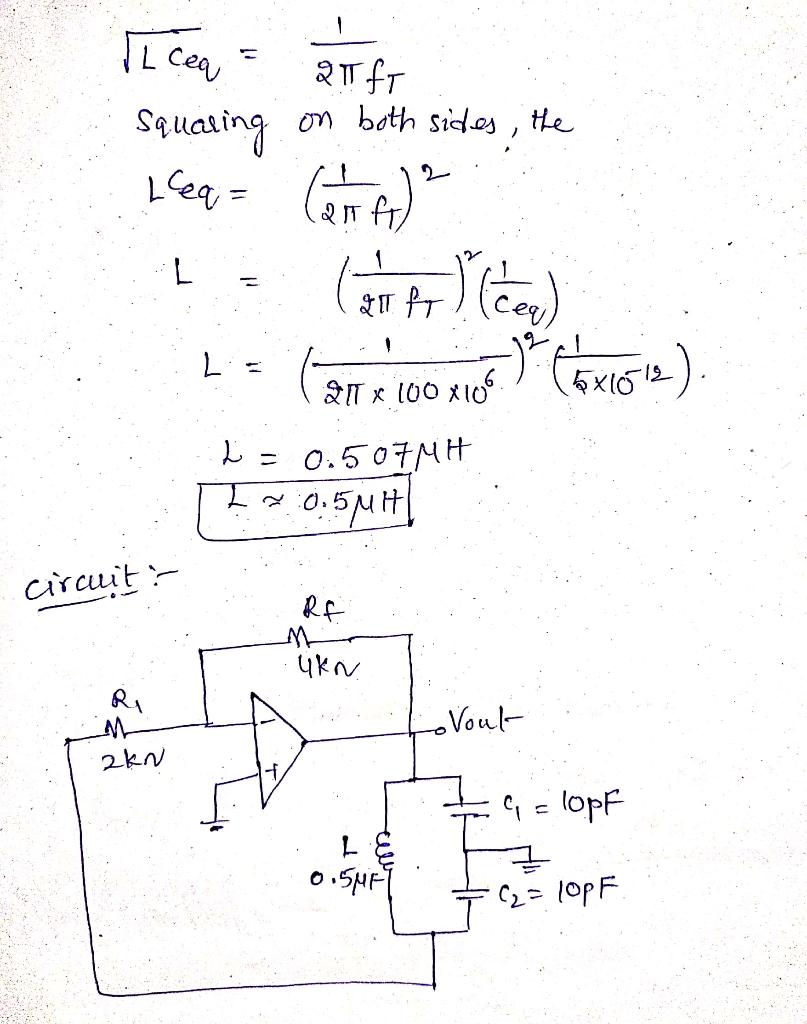Multisim Simulation: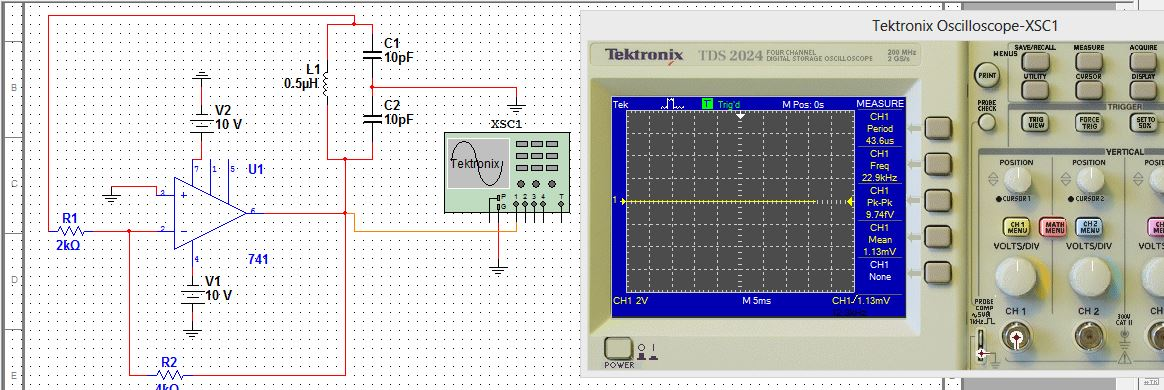#### Earn Coins

Coins can be redeemed for fabulous gifts.

Similar Homework Help Questions
• ### 6. Design a common-base configuration oscillator using BJT in which oscilallate at frequency 2.75 GHz. It scattering pa...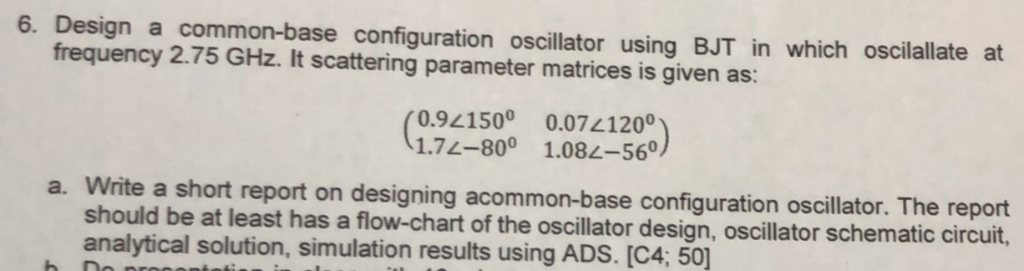6. Design a common-base configuration oscillator using BJT in which oscilallate at frequency 2.75 GHz. It scattering parameter matrices is given as: 0.94500 0.07420° 1.72-800 1.082-560 a. Write a short report on designing acommon-base configuration oscillator. The report should be at least has a flow-chart of the oscillator design, oscillator schematic circuit, analytical solution, simulation results using ADS. [C4; 50] 6. Design a common-base configuration oscillator using BJT in which oscilallate at frequency 2.75 GHz. It scattering parameter matrices is...

• ### In Multisim, rewire your opamp circuit so that it matches the circuit in Figure 2 U1-....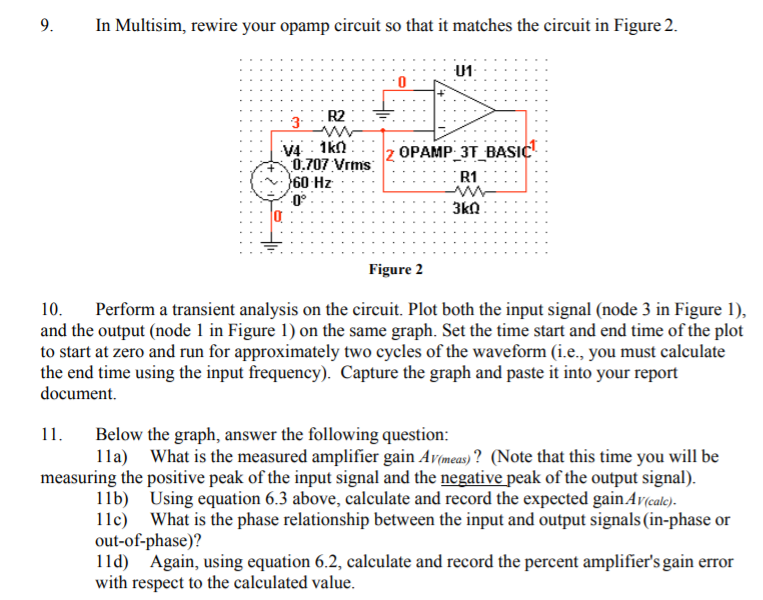In Multisim, rewire your opamp circuit so that it matches the circuit in Figure 2 U1-. V4 1kn 0.707 Vrms z OPAMP 3T BASIC R1 0o Figure 2 10. Perform a transient analysis on the circuit. Plot both the input signal (node 3 in Figure 1), and the output (node 1 in Figure 1) on the same graph. Set the time start and end time of the plot to start at zero and run for approximately two cycles of the...

• ### Using MULTISIM SIMULATION PLEASE!!! using multisim please Reversed Vascu Procedure II: Diode Characteristics: 1. Wire the...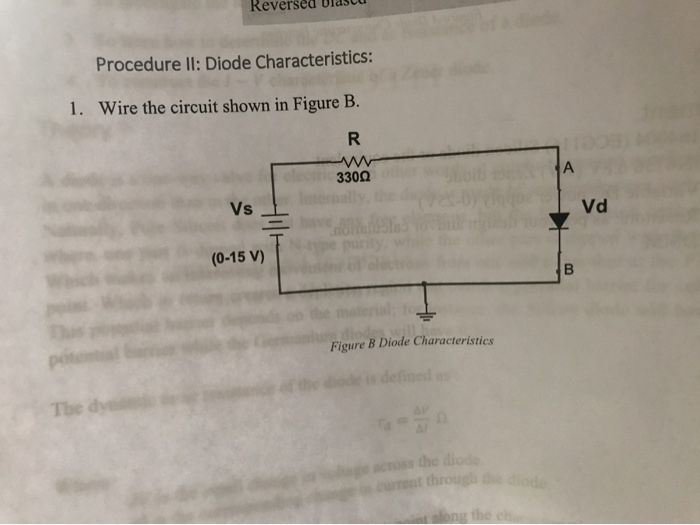Using MULTISIM SIMULATION PLEASE!!! using multisim please Reversed Vascu Procedure II: Diode Characteristics: 1. Wire the circuit shown in Figure B. 3300 LA Vs Vd = (0-15V) Figure B Diode Characteristics pote Mode is defined as The dy to the dio rent through 10 3. Now reverse the diode then adjust the DC supply as shown in Table 2, Record the corresponding current and diode voltage as in step 2. Table 2 Reversed Bias Diode Characteristics Vs (volt) Va la...

• ### Problem # 3 For the summing opamp circuit, assume that the opamp is powered with +15V and-15V. Th...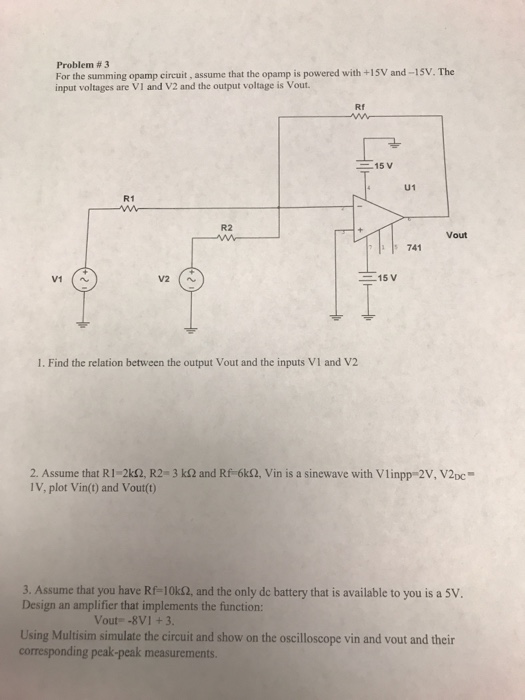Problem # 3 For the summing opamp circuit, assume that the opamp is powered with +15V and-15V. The input voltages are VI and V2 and the output voltage is Vout. Rf 15 V U1 R1 R2 Vout 741 V2 (A -15 V 1. Find the relation between the output Vout and the inputs VI and V2 2. Assume that RI-2kS2, R2-3 ㏀ and Rf-6kS2, Vin is a sinewave with viinpp-2V, V2DC- IV, plot Vin() and Vout(0) 3. Assume that you...

• ### (a) What is the frequency of RTC oscillator and frequency of main oscillator in a Beaglebone...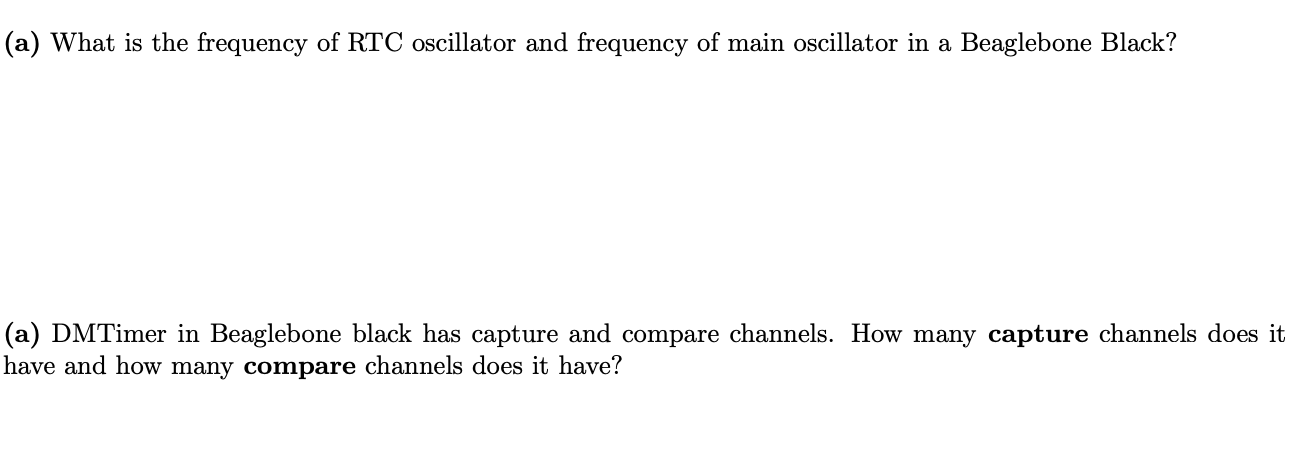(a) What is the frequency of RTC oscillator and frequency of main oscillator in a Beaglebone Black? (a) DMTimer in Beaglebone black has capture and compare channels. How many capture channels does it have and how many compare channels does it have?

• ### 3. When an oscillator with natural frequency wo is driven by another oscillator with a different ...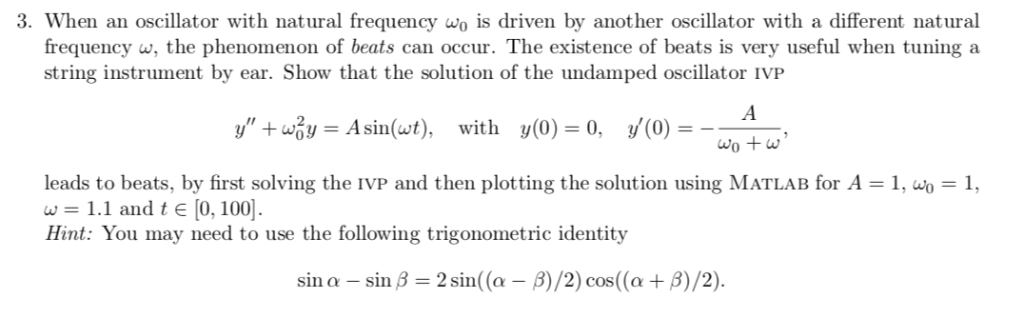3. When an oscillator with natural frequency wo is driven by another oscillator with a different natural frequency w, the phenomenon of beats can occur. The existence of beats is very useful when tuning a string instrument by ear. Show that the solution of the undamped oscillator IVP y', +Hy-Asin(wt), y(0)--, wo+w with y(0)-0, leads to beats, by first solving the IVP and then plotting the solution using MATLAB for A w = 1.1 and t є [0, 1001. 1,...

• ### Simulation For each filter mentioned in the following cases, first simulate the circuit using Multisim. You can get a plot of the transfer function that is called the Bode plot. From the right toolba...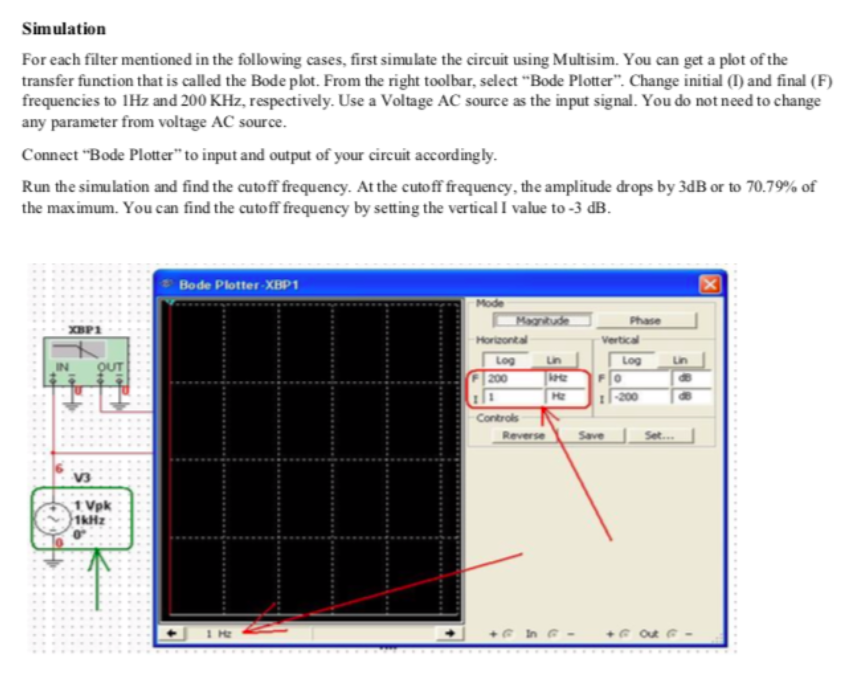Simulation For each filter mentioned in the following cases, first simulate the circuit using Multisim. You can get a plot of the transfer function that is called the Bode plot. From the right toolbar, select "Bode Plotter". Change initial (I) and final (F) frequencies to 1Hz and 200 KHz, respectively. Use a Voltage AC source as the input signal. You do not need to change any parameter from voltage AC source. Connect "Bode Plotter" to input and output of your...

• ### 3) Opamp Frequency Response An opamp comprises 2 voltage gain stages; cach yields a gain of 500. The 3 dB roll- off frequencies of the 2 gain stages are at 40 Hz and 10 MHz, respectively a) What is t...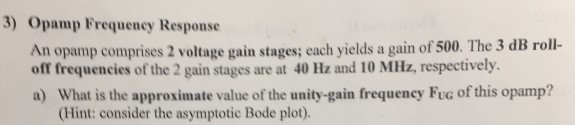3) Opamp Frequency Response An opamp comprises 2 voltage gain stages; cach yields a gain of 500. The 3 dB roll- off frequencies of the 2 gain stages are at 40 Hz and 10 MHz, respectively a) What is the approximate value of the unity-gain frequency FuG of this opamp? (Hint: consider the asymptotic Bode plot). 3) Opamp Frequency Response An opamp comprises 2 voltage gain stages; cach yields a gain of 500. The 3 dB roll- off frequencies of...

• ### can any one help me drow this circuit in multisim and get the simulation output plz...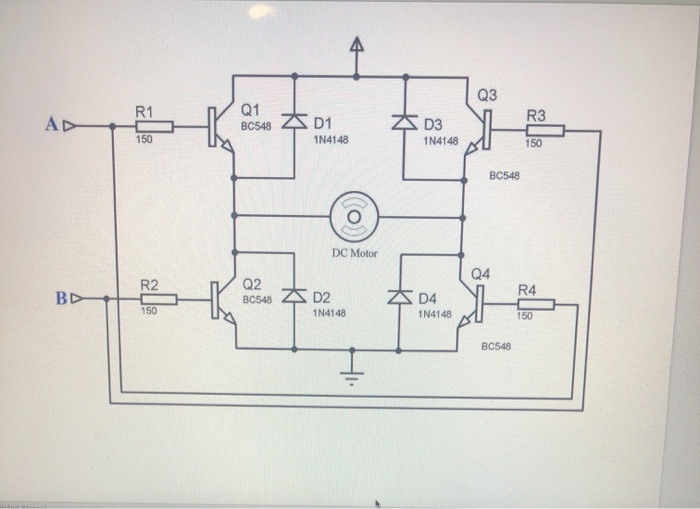can any one help me drow this circuit in multisim and get the simulation output plz Q3 R1 AD Q1 BC548 R3 D1 1N4148 D3 1N4148 150 조 150 BC548 O DC Motor Q4 R2 Q2 BC548 BD R4 4 D2 1N4148 D4 150 1N4148 150 BC548

• ### Circuit for secure telecommunication based on chaotic oscillator: The project involves the simulation and implementation...

Circuit for secure telecommunication based on chaotic oscillator: The project involves the simulation and implementation of a system for communication based on two equal chaotic circuits. Does anyone have any ideas with this theme? Help!